Introduction to using R package: spCP

Use of spCP

This is a brief description of how to use the spCP package within the context of glaucoma progression. In order to fully understand the flexibiltiy of spCP we also load the womblR package, which has some supportive functions. We begin by loading the packages.

library(womblR)
library(spCP)
##
## Attaching package: 'spCP'
## The following object is masked from 'package:womblR':
##
##     diagnostics

In the spCP package there is a longitudinal series of visual fields that we will use to exemplify the statistical models contained in the package. The data object is called VFSeries and has four variables, Visit, DLS, Time and Location. The data object loads automatically; here’s what the data looks like,

##   Visit DLS Time Location
## 1     1  25    0        1
## 2     2  23  126        1
## 3     3  23  238        1
## 4     4  23  406        1
## 5     5  24  504        1
## 6     6  21  588        1

The variable Visit represents the visual field test visit number, DLS the observed outcome variable, differential light sensitvity, Time the time of the visual field test (in days from baseline visit) and Location the spatial location on the visual field that the observation occured. To help illuminate visual field data we can use the PlotVFTimeSeries function from the womblR package. PlotVFTimeSeries is a function that plots the observered visual field data over time at each location on the visual field.

PlotVfTimeSeries(Y = VFSeries$DLS, Location = VFSeries$Location,
Time = VFSeries$Time, main = "Visual field sensitivity time series \n at each location", xlab = "Days from baseline visit", ylab = "Differential light sensitivity (dB)", line.col = 1, line.type = 1, line.reg = FALSE)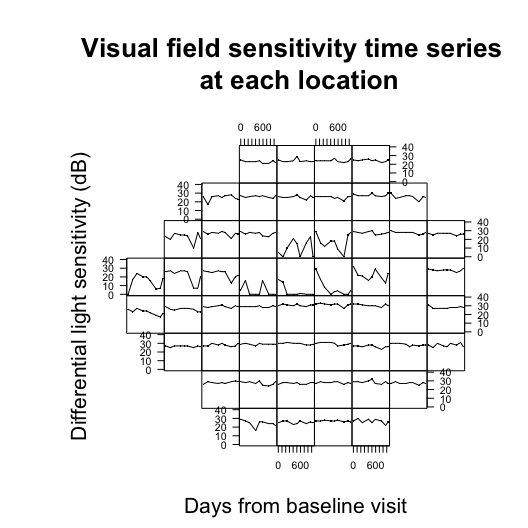The figure above demonstrates the visual field from a Humphrey Field Analyzer-II testing machine, which generates 54 spatial locations (only 52 informative locations, note the 2 blanks spots corresponding to the blind spot). At each visual field test a patient is assessed for vision loss. Format data for spCP We can now begin to think about preparing objects for use in the the spatially varying change point model function (spCP). According to the manual, the observed data Y must be first ordered spatially and then temporally. Furthermore, we will remove all locations that correspond to the natural blind spot (which in the Humphrey Field Analyzer-II correspond to locations 26 and 35). blind_spot <- c(26, 35) # define blind spot VFSeries <- VFSeries[order(VFSeries$Location), ] # sort by location
VFSeries <- VFSeries[order(VFSeries$Visit), ] # sort by visit VFSeries <- VFSeries[!VFSeries$Location %in% blind_spot, ] # remove blind spot locations
Y <- VFSeries$DLS # define observed outcome data Now that we have assigned the observed outcomed Y we move onto the temporal variable Time. For visual field data we define this to be the time from the baseline visit. We obtain the unique days from the baseline visit and scale them to be on the year scale. Time <- unique(VFSeries$Time) / 365 # years since baseline visit
print(Time)
##  0.0000000 0.3452055 0.6520548 1.1123288 1.3808219 1.6109589 2.0712329
##  2.3780822 2.5698630

Our example patient has nine visual field visits and the last visit occured 2.57 years after the baseline visit.

We now specify the adjacency matrix, W, and dissimilarity metric, DM. There are three adjacency matrices for the Humphrey Field Analyzer-II visual field that are supplied by the spCP package, HFAII_Queen, HFAII_QueenHF, and HFAII_Rook. HFAII_Queen and HFAII_QueenHF both define adjacencies as edges and corners (i.e., the movements of a queen in chess), while HFAII_Rook only defines an adjacency as a neighbor that shares an edge (i.e., a rook in chess). The HFAII_QueenHF adjacency matrix does not allow neighbors to share information between the northern and southern hemispheres of the visual field. In this analysis we use the standard queen specification. The adjacency objects are preloaded and contain the blind spot, so we define our adjacency matrix as follows.

W <- HFAII_Queen[-blind_spot, -blind_spot] # visual field adjacency matrix
M <- dim(W) # number of locations

Now we turn our attention to assigning a dissimilarity metric. The dissimilarity metric we use in this data application are the Garway-Heath angles that describe the underlying location that the retinal nerve fibers enter the optic disc. These angles (measured in degrees) are included with spCP in the object GarwayHeath. We create the dissimilarity metric object DM.

DM <- GarwayHeath[-blind_spot] # Garway-Heath angles

The womblR package provides a plotting function PlotAdjacency that can be used to display a dissimilarity metric over the spatial structure of the visual field. We demonstrate it using the Garway-Heath angles.

PlotAdjacency(W = W, DM = DM, zlim = c(0, 180), Visit = NA,
main = "Garway-Heath dissimilarity metric\n across the visual field")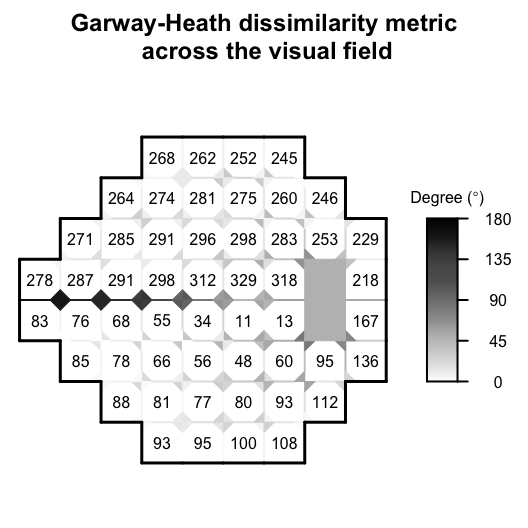Now that we have specified the data objects Y, DM, W and Time, we will customize the objects that characterize Bayesian Markov chain Monte Carlo (MCMC) methods, in particular hyperparameters, starting values, metroplis tuning values and MCMC inputs.

MCMC Characteristics

We begin be specifying the hyperparameters for the model. The parameter $$\alpha$$ is uniformly distributed with lower bound, $$0$$, and upper bound, $$b_{\alpha}$$. The upper bound for $$\alpha$$ cannot be specified arbitrarily since it is important to account for the magnitude of time elapsed. We specify the following upper bound for $$\alpha$$ to dictate a weakly informative prior distribution as specified in Berchuck et al.

pdist <- function(x, y) pmin(abs(x - y), (360 - pmax(x, y) + pmin(x, y))) #Dissimilarity metric distance function (i.e., circumference)
DM_Matrix <- matrix(nrow = M, ncol = M)
for (i in 1:M) {
for (j in 1:M) {
DM_Matrix[i, j] <- pdist(DM[i], DM[j])
}
}
BAlpha <- -log(0.5) / min(DM_Matrix[DM_Matrix > 0])
AAlpha <- 0

Then, we can create a hyperparameters list object, Hypers, that can be used for spCP.

Hypers <- list(Alpha = list(AAlpha = AAlpha, BAlpha = BAlpha),
Sigma = list(Xi = 6, Psi = diag(5)),
Delta = list(Kappa2 = 1000))

Here, $$\delta$$ has a multivariate normal distribution with mean zero and common variance, $$\kappa^2$$, and $$\Sigma$$ has an inverse-Wishart distribution with degrees of freedom $$\xi$$ and scale matrix, $$\Psi$$ (See the help manual for spCP for further details).

Specify a list object, Starting, that contains the starting values for the hyperparameters.

Starting <- list(Sigma = 0.01 * diag(5),
Alpha = mean(c(AAlpha, BAlpha)),
Delta = c(0, 0, 0, 0, 0))

Provide tuning parameters for the metropolis steps in the MCMC sampler.

Tuning <- list(Lambda0Vec = rep(1, M),
Lambda1Vec = rep(1, M),
EtaVec = rep(1, M),
Alpha = 1)

We set Tuning to the default setting of all ones and let the pilot adaptation in the burn-in phase tune the acceptance rates to the appropriate range. Finally, we set the MCMC inputs using the MCMC list object.

MCMC <- list(NBurn = 1000, NSims = 1000, NThin = 2, NPilot = 5)

We specify that our model will run for a burn-in period of 1,000 scans, followed by 1,000 scans after burn-in. In the burn-in period there will be 5 iterations of pilot adaptation evenly spaced out over the period. Finally, the final number of samples to be used for inference will be thinned down to 500 based on the thinning number of 2. We suggest running the sampler 250,000 iterations after burn-in, but in the vignette we are limited by compilation time.

Spatially varying change point model

We have now specified all model objects and are prepared to implement the spCP regression object. To demonstrate the STBDwDM object we will use all of its options, even those that are being used in their default settings.

reg.spCP <- spCP(Y = Y, DM = DM, W = W, Time = Time,
Starting = Starting, Hypers = Hypers, Tuning = Tuning, MCMC = MCMC,
Family = "tobit",
Weights = "continuous",
Distance = "circumference",
Rho = 0.99,
ScaleY = 10,
ScaleDM = 100,
Seed = 54)

## Burn-in progress:  |*************************************************|
## Sampler progress:  0%..  10%..  20%..  30%..  40%..  50%..  60%..  70%..  80%..  90%..  100%..

The first line of arguments are the data objects, Y, DM, W, and Time. These objects must be specified for spCP to run. The second line of objects are the MCMC characteristics objects we defined previously. These objects do not need to be defined for spCP to function, but are provided for the user to custimize the model to their choosing. If they are not provided, defaults are given. Next, we specify that Family be equal to tobit since we know that visual field data is censored. Our distance metric on the visual field is based on the circumference of the optic disc, so we define Distance to be circumference. Finally, we define the following scalar variables, Rho, ScaleY, ScaleDM, and Seed, which are defined in the manual for spCP.

The following are the returned objects from spCP.

names(reg.spCP)
##   "alpha"      "delta"      "sigma"      "beta0"      "beta1"
##   "lambda0"    "lambda1"    "eta"        "theta"      "metropolis"
##  "datobj"     "dataug"     "runtime"

The object reg.spCP contains raw MCMC samples for parameters $$\beta_0(\mathbf{s})$$, $$\beta_1(\mathbf{s})$$, $$\lambda_0(\mathbf{s})$$, $$\lambda_1(\mathbf{s})$$, $$\eta(\mathbf{s})$$, $$\theta(\mathbf{s})$$, $$\delta$$, $$\Sigma$$ and $$\alpha$$, metropolis acceptance rates and final tuning parameters (metropolis) and model runtime (runtime). The objects datobj and dataug can be ignored as they are for later use in secondary functions.

Assessing model convergence

Before analyzing the raw MCMC samples from our model we want to verify that there are no convergence issues. We begin by loading the coda package.

library(coda)

Then we convert the raw spCP MCMC objects to coda package mcmc objects. We look at $$\alpha$$ only for learning purposes.

Alpha <- as.mcmc(reg.spCP$alpha) We begin by checking traceplots of the parameter.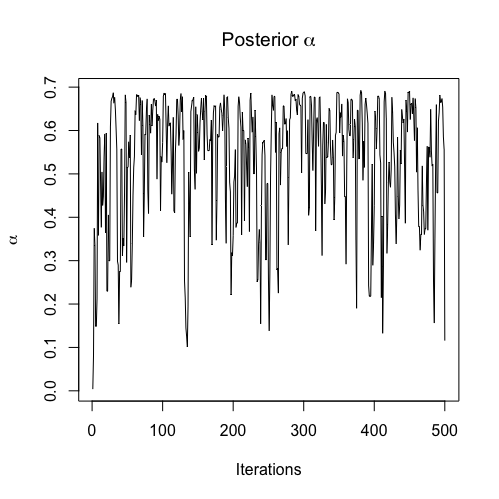From the figure, it is clear that the traceplots exhibit some poor behavior. However, these traceplots are nicely behaved considering the number of iterations the MCMC sampler ran. The traceplots demonstrate that the parameters have converged to their stationary distribution, but still need more samples to rid themselves of autocorrelation. Finally, we present the corresponding test statistics from the Geweke diagnostic test. ## Alpha ## -2.248338 Since none of these test statistics are terribly large in the absolute value there is not strong evidence that our model did not converge. Post model fit analysis Once we have verified that we do not have any convergence issues, we can begin to think about analyzing the raw MCMC samples. A nice summary for spCP is to plot the posterior mean process at each location along with the posterior mean change point. This is possible using the PlotCP in the spCP package. VFSeries$TimeYears <- VFSeries$Time / 365 PlotCP(reg.spCP, VFSeries, dls = "DLS", time = "TimeYears", location = "Location", cp.line = TRUE, cp.ci = TRUE)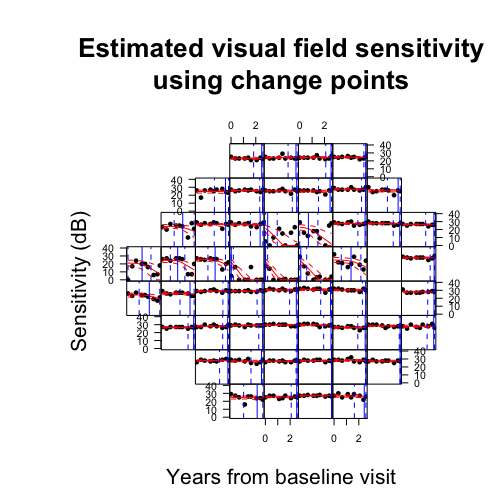Compute diagnostics The diagnostics function in the spCP package can be used to calculate various diagnostic metrics. The function takes in the spCP regression object. Diags <- spCP::diagnostics(reg.spCP, diags = c("dic", "dinf", "waic"), keepDeviance = TRUE) ## Calculating Log-Lik: 0%.. 25%.. 50%.. 75%.. 100%.. Done! ## Calculating PPD: 0%.. 25%.. 50%.. 75%.. 100%.. Done! The diagnostics function calculates diagnostics that depend on both the log-likelihood and posterior predictive distribtuion. So, if any of these diagnostics are specified, one or both of these must be sampled from. The keepDeviance and keepPPD indicate whether or not these distributions should be saved for the user. We indicate that we would like the output to be saved for the log-likelihood (i.e., deviance). We explore the output by looking at the traceplot of the deviance. Deviance <- as.mcmc(Diags$deviance)
traceplot(Deviance, ylab = "Deviance", main = "Posterior Deviance")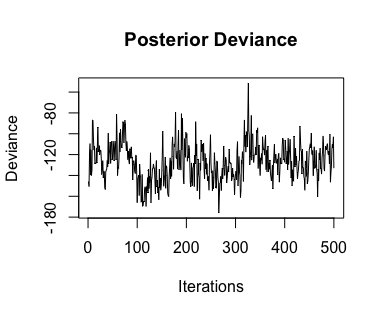This distribution has possible convergence issues, however this is not concerning given the number of MCMC iterations run.

print(Diags)
##       dic        pd
## -43.02116  84.06801
##         p         g      dinf
##  10180.88 247611.10 257791.98
##     waic   p_waic     lppd p_waic_1
## -8.55283 77.61843 81.89485 36.70052

Future prediction

The spCP package provides the predict.spCP function for sampling from the posterior predictive distribution at future time points of the observed data. This is different from the posterior predictive distribution obtained from the diagnostics function, because that distribution is for the observed time points and is automatically obtained given the posterior samples from spCP. We begin by specifying the future time points we want to predict as 50 and 100 days past the most recent visit.

Nu <- length(Time) # calculate number of visits
NewTimes <- Time[Nu] + c(50, 100) / 365

Then, we use predict.spCP to calculate the future posterior predictive distribution.

Predictions <- predict(reg.spCP, NewTimes)
## Future Predictions: 0%.. 25%.. 50%.. 75%.. 100%.. Done!

We can see that predict.spCP returns a list containing a matrix of predictions corresponding to each future time point. The name of each matrix is the numeric time point for each future visit.

names(Predictions)
##  "2.70684931506849" "2.84383561643836"

You can plot a heat map representation of the posterior distribution of the change points using the function PlotSensitivity from womblR.

CPProbs <- apply(reg.spCP\$eta, 2, function(x) mean(x < Time[Nu]))
PlotSensitivity(Y = CPProbs,
main = "Probability of an observed \n change point",
legend.lab = expression(paste("Pr[", eta, "(s)] < ", t)), legend.round = 2,
bins = 250, border = FALSE)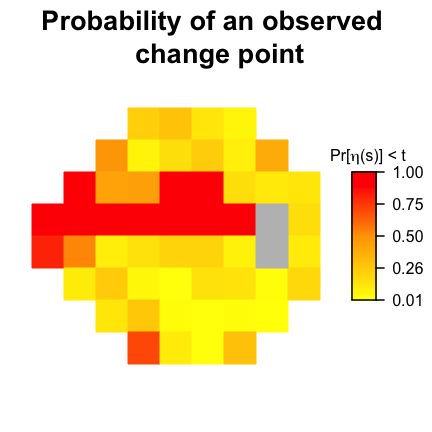This figure shows the posterior probabiltiy that a change point has occured in the follow-up period over the visual field. The PlotSensitivity function can be used for plotting any observations on the visual field surface.